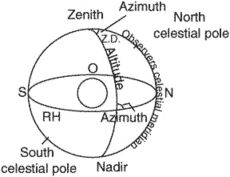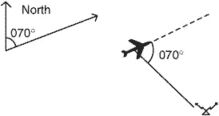azimuth angle

Also found in: Dictionary, Acronyms.
Related to azimuth angle: Zenith Angle

azimuth angle

[′az·ə·məth ′aŋ·gəl]
(engineering)
An angle in triangulation or in traverse through which the computation of azimuth is carried.

azimuth angle (Z)Azimuth angles in both these two cases are 070°.
i. The arc of the horizon between the observer's meridian and the vertical circle of an object, measured either from the north or south, to the right or clock-wise, or to the left or counterclock-wise, through 90° or 180°. It must be labeled north or south (as a prefix) and east or west (as a prefix) to indicate the direction of measurement.
ii. An angle measured clock-wise in the horizontal plane between a reference direction and any other line.
References in periodicals archive ?
By means of this information, the azimuth angle of the sound source can be calculated easily.
theta](k) and [phi](k) are the pitching and azimuth angles, respectively, which can be detected by the aircraft radar warning device .
From Fig 5, it is observed that the effect of PPD is almost constant for a range of azimuth angle from AY=0 to AY=10, or adding some tolerance it goes up to AY =20.
2 and 3, respectively, plot the elevation angle and azimuth angle estimated by the proposed method, at different signal-to-noise ration (SNR) levels.
When we are only concerned with the estimation of the azimuth angle, the optimization of one-dimensional cost function using exhaustive grid search even with small search step is quite feasible.
Input azimuth angle of arrival for desired signal =40[degrees] Input elevation angle of arrival for desired signal =20[degrees]
Hearing aids are tested in/on the right ear of the manikin, which is mounted on a turntable that rotates the manikin in the horizontal plane to adjust the azimuth angle.
RTM 3D gathers contain full-azimuth information such as subsurface reflection angle and azimuth angle.
RMS value of the n samples length output signal and j azimuth angle is:
facade PV collector), the tilt angle would remain 90[degrees] by tracking and the deflection angle would be equal to the collector azimuth angle
An azimuth angle will have one of these reference points, and it will be a continuous angle turned either to the right (cw) or to the left (ccw).

Site: Follow: Share:
Open / Close